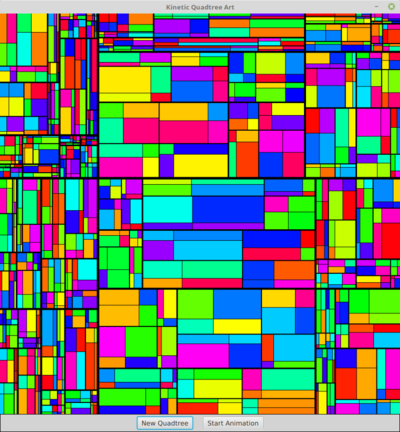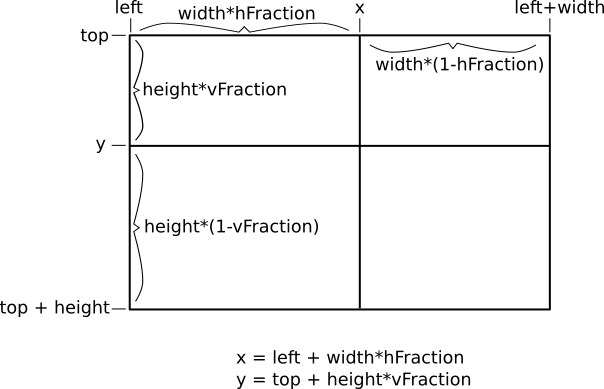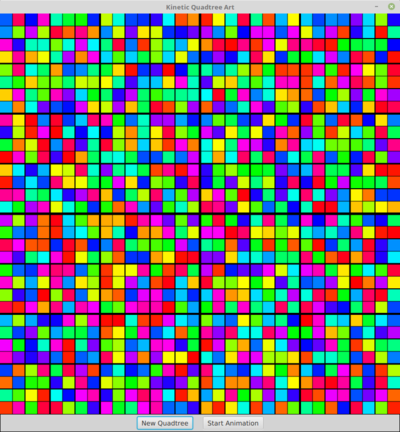## CPSC 225, Spring 2019 Lab 7: Trees

This week's lab is about recursive processing of trees. A warm-up exercises asks you to write three short recursive methods that operate on standard binary trees. The main part of the lab asks you to work with quadtrees, in which non-leaf nodes have four children instead of two. You will use quadtrees to implement a kind of recursive kinetic art. When writing the recursive methods for this lab, keep in mind that if you are writing a lot of code, then you are probably writing too much! Here's one frame from the quadtree animation, shown at reduced size:You will need the two files from /classes/cs225/lab7_files: SimpleTree.java and QuadTreeArt.java. The file QuadTreeArt.java will have syntax errors until you write the class that it depends on, QuadTreeNode.

Your work for this lab is due next Tuesday, as usual. You should submit the files SimpleTree.java, QuadTreeArt.java, and QuadTreeNode.java to your homework folder, inside a folder named lab7.

### Warm-up: Simple Tree Demo

The file SimpleTree.java is an almost complete program that builds three binary sort trees and prints out some information about each tree. However, three of the methods that are supposed to compute information about the trees are left incomplete. You should complete the three recursive methods: countNodes(), countLeaves(), and treeHeight(). Read the comments on these methods to find out what they should do.

Several methods that we looked at in class are already complete in the file, including treeSum(), which computes the sum of all items in a tree. Note that none of the methods that you need to complete should be much longer than a half-dozen lines.

The first two trees that are created in the program are made by inserting the elements from an array into a tree that is initially empty. You can do the insertion by hand and draw those two trees, and use the result to make sure that your methods are computing the correct answers.

### Kinetic Tree Art

The file QuadTreeArt.java is the main program for the main assignment of the lab. The program makes use of a class named QuadTreeNode, which is not yet defined. Your assignment is to create a new file named QuadTreeNode.java to define that class. The class will have to provide the recursive constructor and two recursive methods that are needed by QuadTreeArt. You can start by creating the constructor and methods as "stubs," which have empty definitions, to get rid of the syntax errors in QuadTreeArt. You will not need to make any changes to QuadTreeArt.java (but you might make some changes to it, if you decide to extend the program as suggested at the end of this web page).

The completed QuadTreeArt program was demonstrated in class. It displays animated art based on "quadtrees." In a quadtree, a node that is not a leaf has four child trees. In the quad trees that we use in this lab, every node in a quad tree represents an image that can be drawn in a rectangular area. For a leaf node, the picture is just a rectangle filled with a single color. For a non-leaf node, the rectangular picture is divided into four rectangular pieces or "quadrants," which are referred to as upperLeft, upperRight, lowerLeft, and lowerRight:Each rectangular quadrant corresponds to one of the child trees of the node, and the quadrant will be filled with the picture represented by the corresponding child tree. Note that the four quadrants are not the same size. When the art is animated, the horizontal and vertical dividing lines between the quadrants will move, and the picture in each quadrant will adjust its size to fit.

A quadtree contains two kinds of node, leaf nodes and non-leaf nodes. The QuadTreeNode class can use an instance variable

```boolean isLeaf;   // true, if this node is a leaf
```

to record whether it is a leaf node or a non-leaf node. Some of the other instance variables will be used only for leaf nodes, while other instance variables will be used only for non-leaf nodes. (An alternative would be to define two different classes to represent leaf and non-leaf nodes, and to make both of those classes subclasses of an abstract QuadTreeNode class. However, that does complicate things and I am not asking you to do that.)

When a non-leaf node wants to draw its picture in a given rectangle, it has to divide that rectangle into four quadrants. The first difficulty is that the node has to be able to draw its picture in a rectangle of any given size. The second difficulty is that the horizontal and vertical dividing lines between the four quadrants will have to move. To make this possible, the node needs two instance variables

```double hFraction; // Fraction of total width occupied by left quadrants.
double vFraction; // Fraction of total height occupied by top quadrants.
```

To keep the sizes of the quadrants reasonable, the values of hFraction and vFraction should always be between 0.2 and 0.8.

Suppose that the node wants to draw in a rectangle that has its top left corner at (left,top), has horizontal size width and has vertical size height. Then the sizes and locations of the four quadrants can be computed according to this diagram:The values from this diagram can be used by a non-leaf node to draw its four child trees.

You will need to define a constructor for the QuadTreeNode class that has one parameter of type int. The parameter specifies the tree-height of the quadtree that is being constructed. A tree of tree-height zero is a leaf; a leaf should choose its color at random. A tree of tree-height greater than zero is a non-leaf node, which has four child nodes whose tree-heights are one less than the tree-height of the node that is being constructed. For a non-leaf node, the constructor can assign the value 0.5 to both the hFraction and vFraction instance variables. That will give a tree that initially produces a picture like this one:The QuadTreeNode contains an instance method

```public void draw( GraphicsContext g,
double left, double top,
double rectWidth, double rectHeight )
```

This draw method should draw the image represented by the node in the rectangle with upper left corner at (left,top) and with the given width and height. To make the image look nice, you should stroke the horizontal and vertical dividing lines that separate the quadrants in a non-tree node.

Note that to test your draw() method before you start working on animation, you might want to assign random values in the range 0.2 to 0.8 to hFraction and vFraction. However, in the end, the animation will work best if you initialize those values to 0.5.

Finally, you have to worry about the animation. The QuadTreeNode class defines a method

```public void update(int frameNumber)
```

This method is called just before each frame of the animation is drawn. Its purpose is to update the values of the variables hFraction and vFraction in a non-leaf node (and of course in all of its child trees). Nothing needs to be done to update a leaf node (unless you wanted to animate the color, which might be an interesting effect.) The question is, how to set the values of hFraction and vFraction? Each of these variables should oscillate between their minimum value (0.2) and their maximum value (0.8). They need to oscillate at different, randomly chosen rates. There are several ways to make the value of a variable go through a loop as the frameNumber increases. The one that I like for this project uses the mathematical function sin(x), which gives a nice, smooth oscillating motion. Suppose that you want the value of a variable to loop between 0.2 and 0.8 over the course of P frames. (The "P" stands for "period" and is referred to as the period of the loop.) Then in each frame, the value of the variable can be set to

```0.5  +  0.3 * Math.sin( 2*Math.PI * frameNumber / P )
```

When a non-leaf node is constructed, you just need to randomly select the period that will be used for hFraction, and randomly select another period to be used for vFraction. Keep in mind that the period represents the number of frames that it takes for the loop to repeat, and that there are 60 frames per second. You will probably have to experiment to get reasonable speeds.

I could suggest a few improvements to the program. These improvements are not required. For one thing, I think the program looks nicer if the lines that separate the quadrants in a node's picture have different linewidths, depending on how deep the node is in the tree. In fact, the quadtree images on this page use that effect. To implement this in my program, I save the tree-height variable from the constructor that created the node in an instance variable, and I use that value as the linewidth for the lines in the draw() method.

The second improvement requires you to modify QuadTreeArt.java to give the user the option of making art in which the colors of the leaf nodes are shades of gray. You can easily add a CheckBox to the strip of controls along the bottom of the window. When a new quadtree is constructed, the setting of the CheckBox can be used to determine whether the new tree should use color or gray. To implement this, you will need to add a boolean parameter to the QuadTreeNode constructor to tell it whether to use color or gray.

And one more tiny improvement: I think that the animation looks better when it is starting if some of the periods for hFraction and vFraction are negative numbers. To implement that, you can select a positive period at random then, if the value of a random number is less than 0.5, multiply the selected period by -1. (Without this modification, all of the dividing lines start out moving down or to the right when the animation begins; a negative period makes a line start out moving up or to the left.)